## Objective:

1. Estimate survival function estimate for given time-to-event data. Here, event considered is a binary event.
2. Compare survival curve estimates for 2 segments of data.

## Solution:

We can use survival package to accomplish this.

1. Surv function to get the data in the right format.
2. which will then be passed tp survfit function to compute simple Kaplan-Meier estimator.
3. survdiff function to test if difference between 2 survival functions is significant.

## Code:

# install.packages("OIsurv")
# install.packages("survsim")
# install.packages("broom")
library(OIsurv)
## Loading required package: survival
## Loading required package: KMsurv
# library(help=KMsurv)
library(dplyr)
##
## Attaching package: 'dplyr'
##
## The following object is masked from 'package:stats':
##
##     filter
##
## The following objects are masked from 'package:base':
##
##     intersect, setdiff, setequal, union
library(broom)
library(ggplot2)
library(survsim)
## Loading required package: eha
## Loading required package: statmod
# Real dataset (From KMSurv package)
# help(tongue)
data(tongue)
head(tongue)
##   type time delta
## 1    1    1     1
## 2    1    3     1
## 3    1    3     1
## 4    1    4     1
## 5    1   10     1
## 6    1   13     1
summary(tongue)
##       type           time            delta
##  Min.   :1.00   Min.   :  1.00   Min.   :0.0000
##  1st Qu.:1.00   1st Qu.: 23.75   1st Qu.:0.0000
##  Median :1.00   Median : 69.50   Median :1.0000
##  Mean   :1.35   Mean   : 73.83   Mean   :0.6625
##  3rd Qu.:2.00   3rd Qu.:101.75   3rd Qu.:1.0000
##  Max.   :2.00   Max.   :400.00   Max.   :1.0000

This dataset is about 80 male subjects who were diagnosed with one of the 2 types of cancer of the tongue. Time tp death is expressed in weeks. More info on this can be found from the package documentation for this dataset using help(tongue).

# Analyzing just one type of tumor (Aneuploid Tumor)
tongue2 = tongue %>%
filter(type == 1)

# Converting into a Surv object
tongue2_surv <- Surv(tongue2$time, tongue2$delta)

# Getting KM estimator
tongue2_survfit = survfit(tongue2_surv ~ 1)
plot(tongue2_survfit)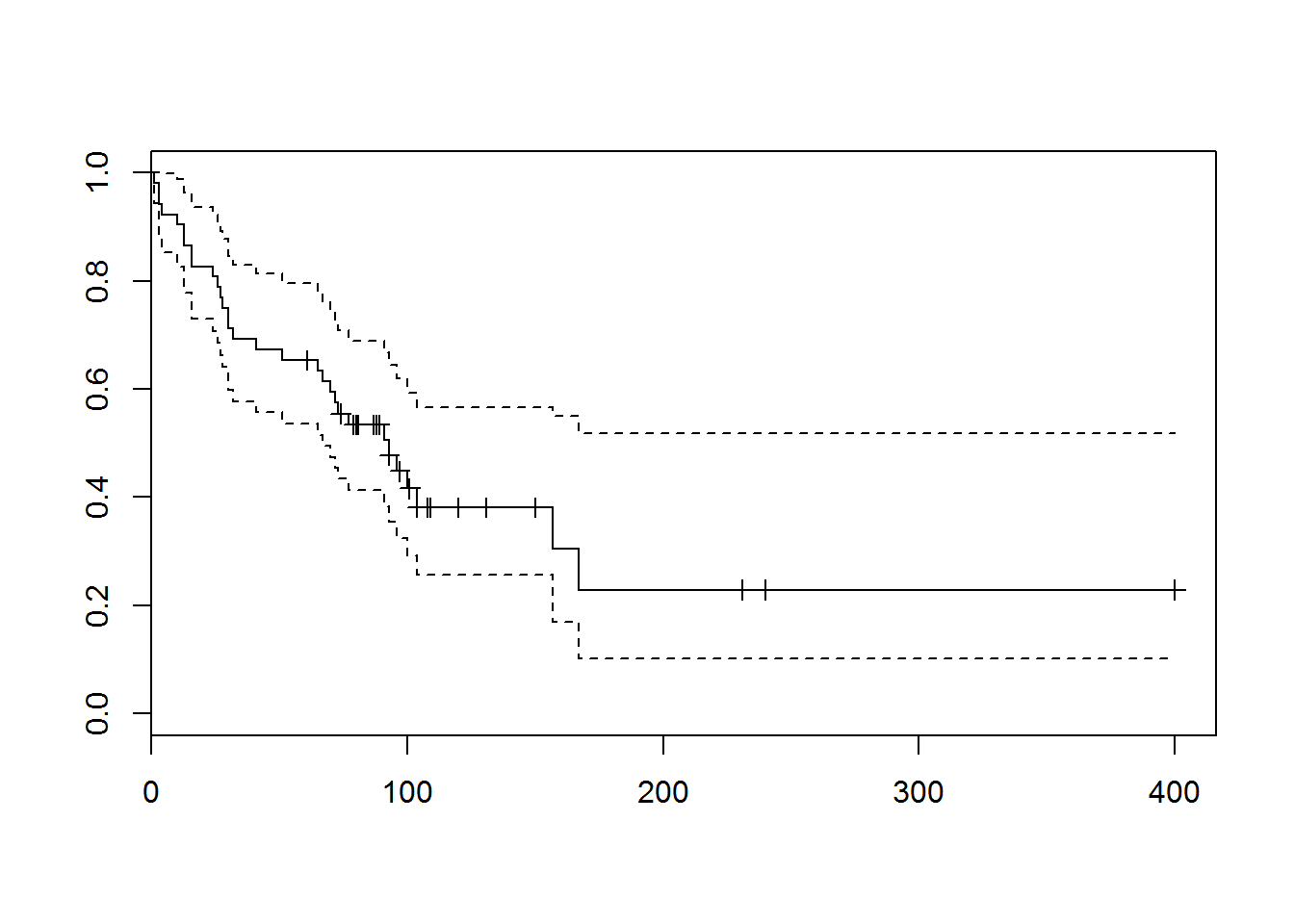• The above plot shows Kaplan Meier estimator of the survival function (solid line) along with the confidence interval (dotted lines).
• The + signs represent censoring i.e. subjects for whom we don’t have any readings available after that week. The only thing we know is the subject didn’t die until that point in time.
glance(tongue2_survfit)
##   records n.max n.start events median conf.low conf.high
## 1      52    52      52     31     93       67        NA

The median value of 93 implies, 50% of the subjects died within 93 weeks of diagnosis.

# Graphically Comparing KM estimator for 2 tumors
tongue_survfit = survfit(Surv(time = time, event = delta) ~ type, data = tongue)
plot(tongue_survfit, lty = 2:3, xlab = "weeks", ylab = "Proporation Survival")
legend(100, .8, c("Aneuploid", "Diploid"), lty = 2:3)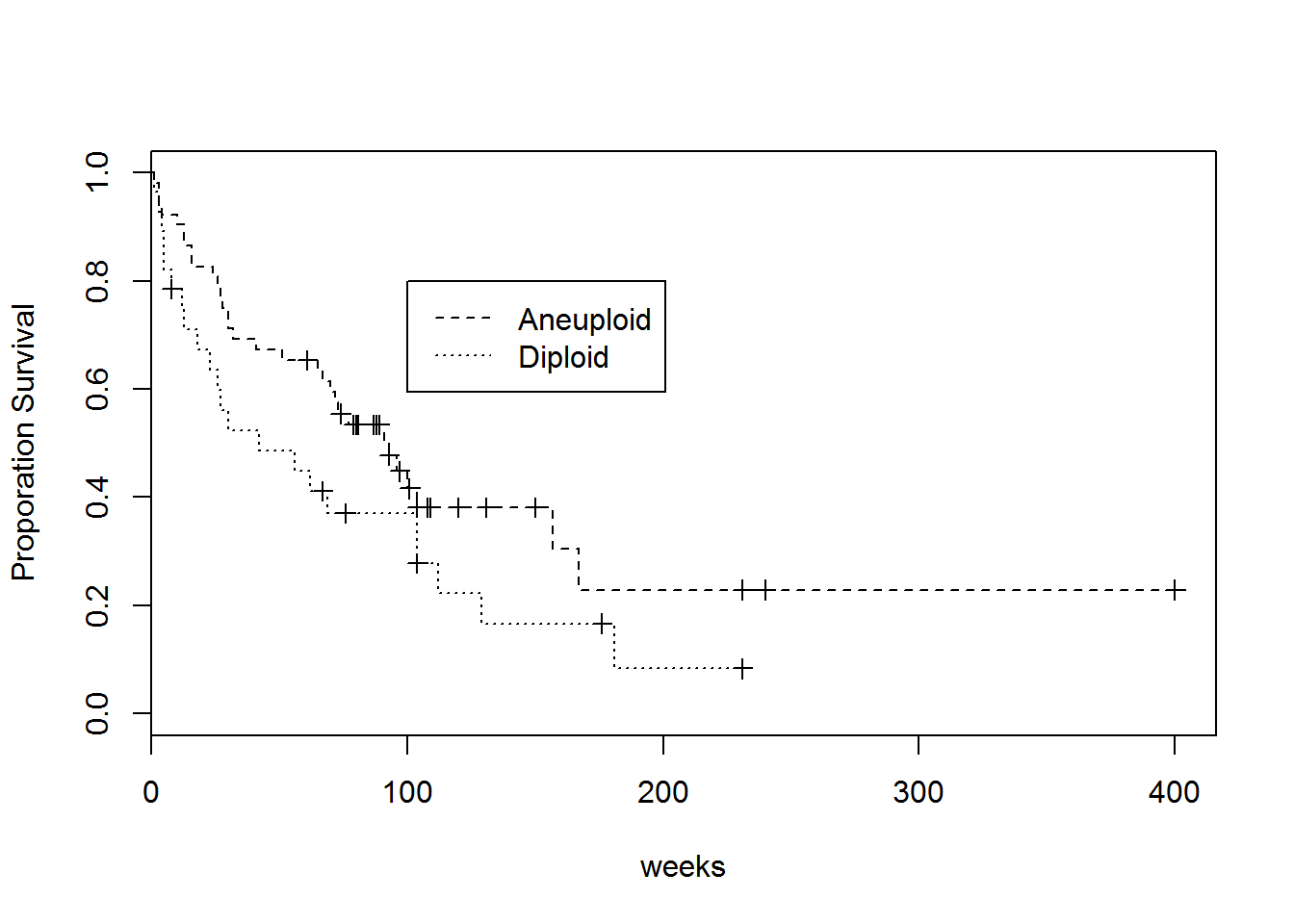• The above plot compares the KM estimator for the survival functions for the 2 types of cancer.
• Shown below is a ggplot2 version of the same plot alongwith the confidence intervals (shaded).
• Different symbols (circles, triangles, etc.) represent no. of observations censored at that time.
• since the confidence interval for type=2 strata slightly overlaps the type=1 strata, we can’t really say if the survival functions are different for the 2 types of cancer. Let’s resort to statistical tests then.
# ggplot2 version of the plot
tongue_tidy = tidy(tongue_survfit)
mx = max(tongue_tidy$n.censor) ggplot(tongue_tidy, aes(time, estimate, fill = strata)) + geom_line() + geom_point(aes(shape = as.factor(n.censor)), size = 3) + scale_shape_manual(values=c(NA, 1:mx))+ geom_ribbon(aes(ymin=conf.low, ymax=conf.high), alpha=.25) + xlab("weeks") + ylab("Proportion Survival") ## Warning in loop_apply(n, do.ply): Removed 43 rows containing missing values ## (geom_point).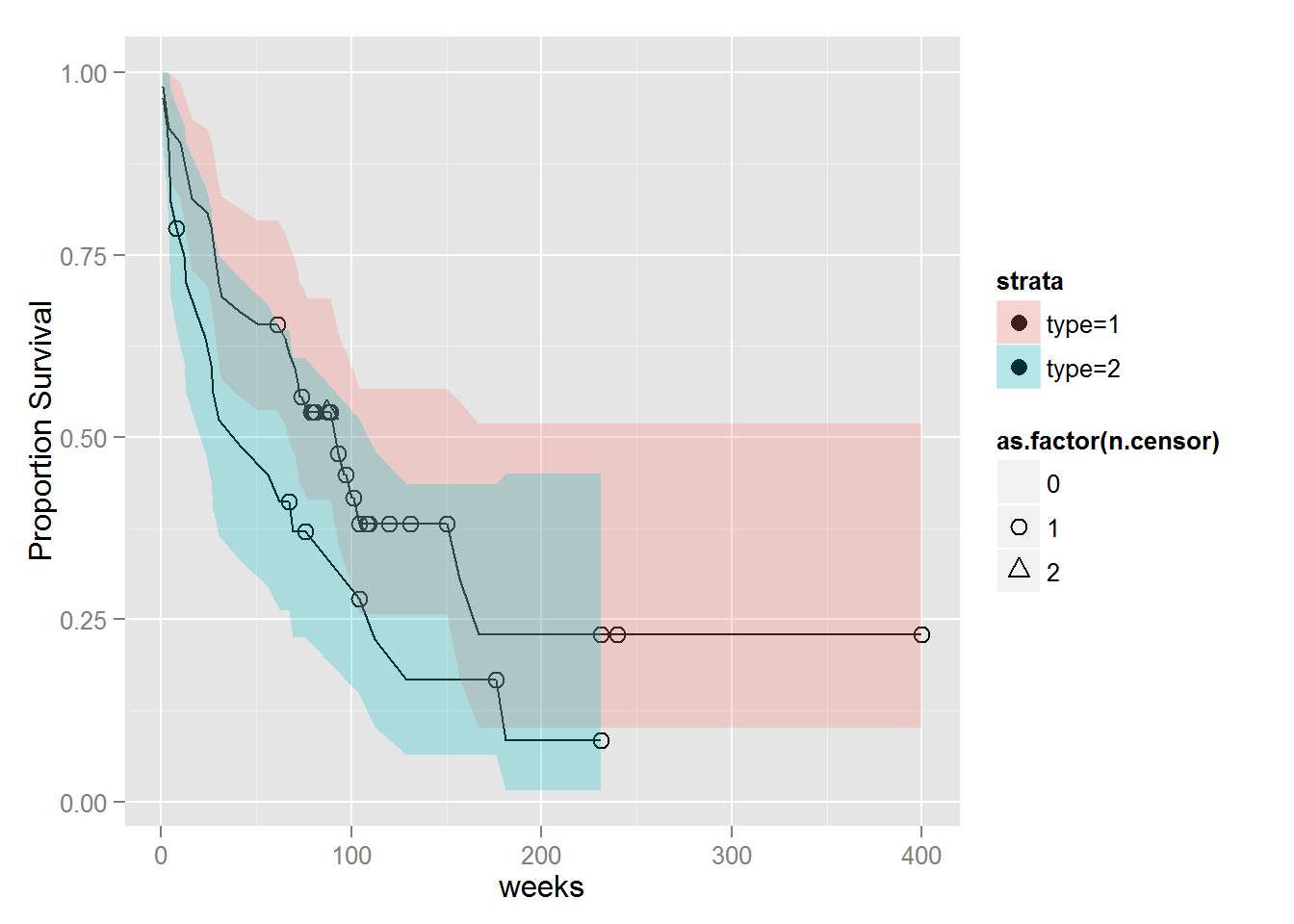We can compare the survival curves using various statistical tests using survdiff function. Apart from the usual formula and data arguments it takes rho is an argument. Setting different values of rho leads to different kinds of test. In general - • rho > 0 implies higher weight is given to initial part of the survival curve • rho < 0 implies higher weight is given to latter part of the survival curve Depending on the value of rho we can get different results as can be seen in the below example. # Statistical tests for comparison survdiff(Surv(time = time, event = delta) ~ type, data = tongue, rho = 0) # log-rank, default ## Call: ## survdiff(formula = Surv(time = time, event = delta) ~ type, data = tongue, ## rho = 0) ## ## N Observed Expected (O-E)^2/E (O-E)^2/V ## type=1 52 31 36.6 0.843 2.79 ## type=2 28 22 16.4 1.873 2.79 ## ## Chisq= 2.8 on 1 degrees of freedom, p= 0.0949 survdiff(Surv(time = time, event = delta) ~ type, data = tongue, rho = 1)  ## Call: ## survdiff(formula = Surv(time = time, event = delta) ~ type, data = tongue, ## rho = 1) ## ## N Observed Expected (O-E)^2/E (O-E)^2/V ## type=1 52 20.2 24.4 0.731 3.3 ## type=2 28 15.1 10.9 1.643 3.3 ## ## Chisq= 3.3 on 1 degrees of freedom, p= 0.0694 It outputs a chi-squared statistic as well as p-value which can be used to determine whether the difference is significant at certain significance level. ### Simulating a dataset using simple.surv.sim function from survsim package. • Time-to-event: Assumed to be following weibull distribution with p = 1.5 i.e. the mode of the time to event is closer to median then for exponential distribution (p = 1) • Time-to-censoring: Assumed to be following weibull distribution with p = 10 i.e. censoring is happening mostly in the latter part of the survival curve. • Covariate: 1 Binary covariate with p = .7 and beta of 0.6. (In the following post I’ll try to reverse-engineer this beta using coxph or other similar models) Following is the same analysis using this simulated dataset. Try and see if you can make sense of code and the output. set.seed(2365) d1 = simple.surv.sim(n = 500, foltime = 1000, dist.ev = 'weibull', anc.ev = 1.5, beta0.ev = 2, # event dist - weibull with p = 1.5 dist.cens = 'weibull', anc.cens = 10, beta0.cens = 2.01, # censoring dist - weibull with p = 10 # z = list(c("weibull", 1)), #assuming independent observations i.e. no within subject correlation beta = list(c(.6)), x = list(c("bern", .7)) # 1 binary covariate ) # View(d1) summary(d1) ## ## Number of subjects at risk ## ---------------------------- ## sub.risk ## 500 ## ## ## Number of events ## ---------------------------- ## num.events ## 186 ## ## ## Proportion of subjects with event ## ---------------------------- ## mean.ep.sub ## 0.372 ## ## ## Total time of follow-up ## ---------------------------- ## foltime ## 2964.863 ## ## ## Time of follow-up (median) ## ---------------------------- ## med.foltime ## 6.612405 ## ## ## Density of incidence ## ---------------------------- ## dens.incid ## 0.06273477 summary(as.data.frame(d1)) ## nid status start stop z ## Min. : 1.0 Min. :0.000 Min. :0 Min. :0.1452 Min. :1 ## 1st Qu.:125.8 1st Qu.:0.000 1st Qu.:0 1st Qu.:4.8752 1st Qu.:1 ## Median :250.5 Median :0.000 Median :0 Median :6.6124 Median :1 ## Mean :250.5 Mean :0.372 Mean :0 Mean :5.9297 Mean :1 ## 3rd Qu.:375.2 3rd Qu.:1.000 3rd Qu.:0 3rd Qu.:7.4102 3rd Qu.:1 ## Max. :500.0 Max. :1.000 Max. :0 Max. :9.1243 Max. :1 ## x ## Min. :0.000 ## 1st Qu.:0.000 ## Median :1.000 ## Mean :0.714 ## 3rd Qu.:1.000 ## Max. :1.000 d1_survfit = survfit(Surv(time = start, time2 = stop, event = status) ~ x, data = d1) d1_survfit = survfit(Surv(time = stop, event = status) ~ x, data = d1) # same as above plot(d1_survfit, lty = 2:3, xlab = "time", ylab = "Proporation Survival") legend(100, .8, c("x=0", "x=1"), lty = 2:3)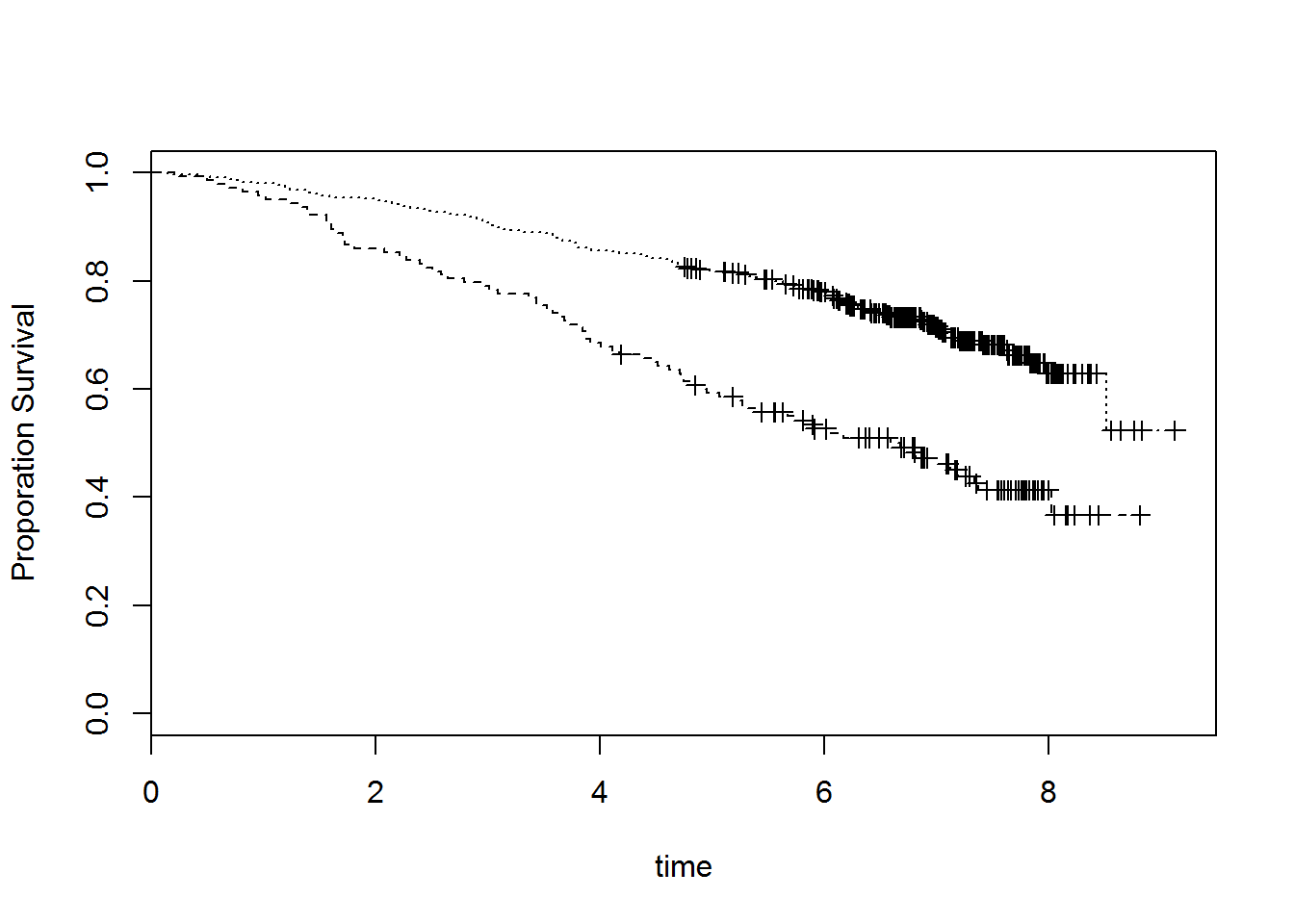# ggplot2 version of the plot d1_tidy = tidy(d1_survfit) mx = max(d1_tidy$n.censor)
ggplot(d1_tidy, aes(time, estimate, fill = strata)) +
geom_line() +
geom_point(aes(shape = as.factor(n.censor)), size = 2) +
scale_shape_manual(values=c(NA, 1:mx))+
geom_ribbon(aes(ymin=conf.low, ymax=conf.high), alpha=.25) +
xlab("time") +
ylab("Proportion Survival")
## Warning in loop_apply(n, do.ply): Removed 186 rows containing missing
## values (geom_point).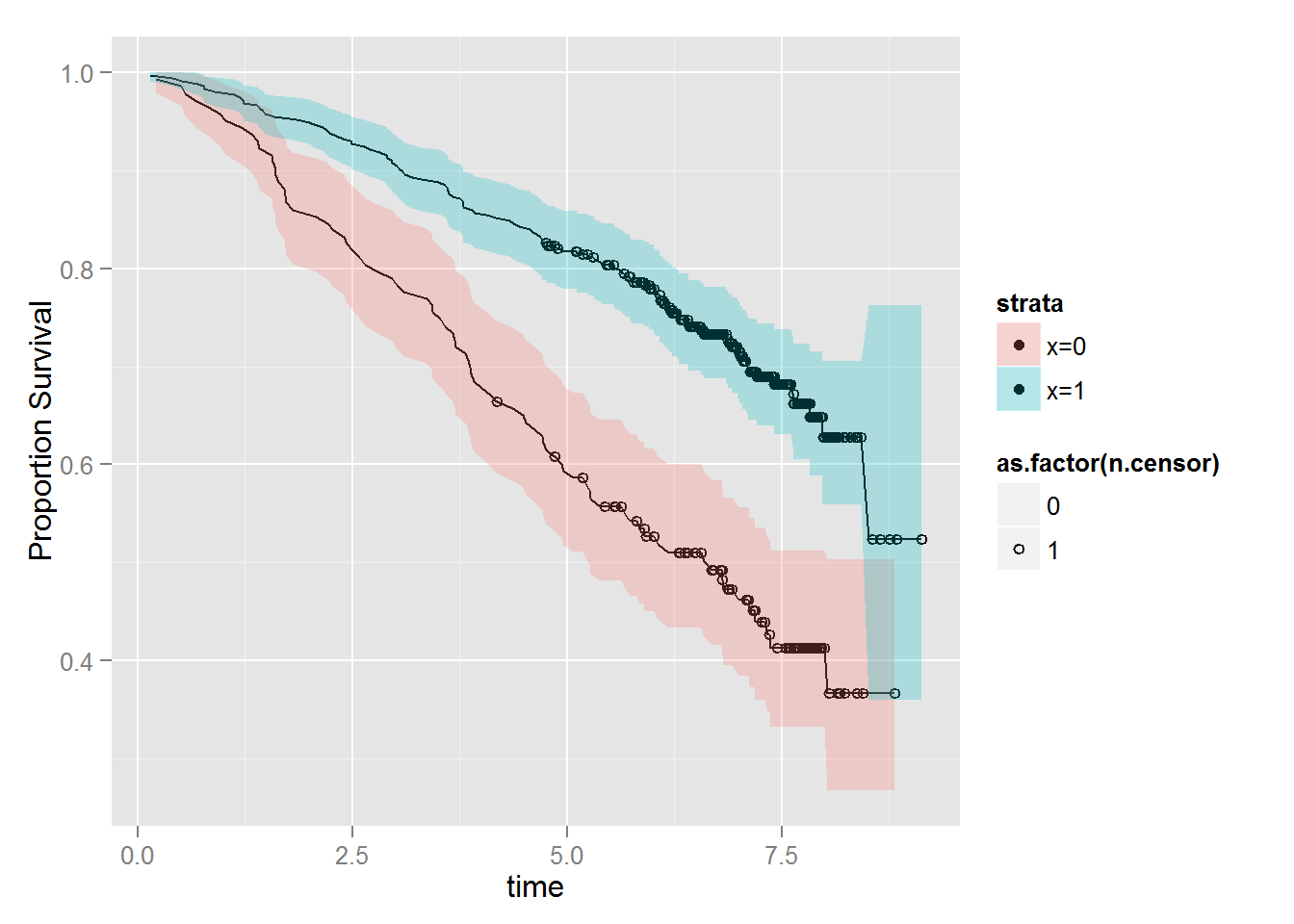# Statistical tests for comparison
survdiff(Surv(time = stop, event = status) ~ x, data = d1, rho = 0) 
## Call:
## survdiff(formula = Surv(time = stop, event = status) ~ x, data = d1,
##     rho = 0)
##
##       N Observed Expected (O-E)^2/E (O-E)^2/V
## x=0 143       79     45.7     24.30      32.3
## x=1 357      107    140.3      7.91      32.3
##
##  Chisq= 32.3  on 1 degrees of freedom, p= 1.31e-08